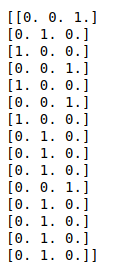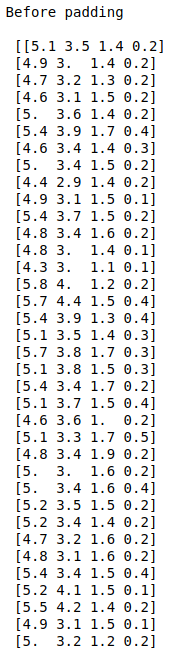• #5, First Floor, 4th Street Dr. Subbarayan Nagar Kodambakkam, Chennai-600 024 Landmark : Samiyar Madam
• pro@slogix.in
• +91- 81240 01111

### How to do Sequence padding and one-hot encoding of independent variables using python?

###### Description

To encode the target variable and do padding for independent variables using keras.

Iris data set.

#### Output

Padded sequence of input data points.
Encoded target variables.

###### Process

Take features and target variables as an array.

Because in deep learning with keras everything should be in array.

Initialize sequence padding and one-hot encoder constructor from keras library.

Fit the data into the constructor.

Transform the independent and target variables.

###### Sample Code

#import necessary libraries
import pandas as pd
import numpy as np
import keras
from keras.preprocessing import sequence
from keras.utils import np_utils
from sklearn.model_selection import train_test_split

#load the sample data from csv file

#Make it as a data frame
df = pd.DataFrame(data)

#feature selection
X = np.array(df.iloc[:,0:4])
y = np.array(df.iloc[:,5])

#Split the data into train and testing
X_train, X_test, Y_train, Y_test = train_test_split(X, y, test_size=0.1, random_state=0)